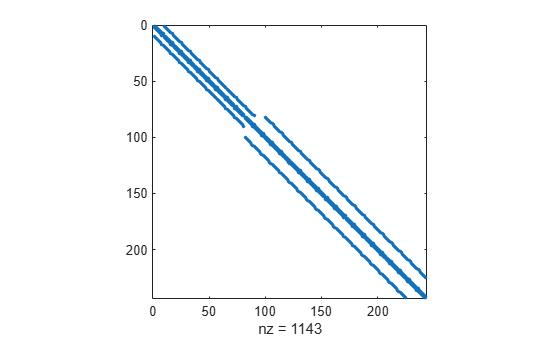# nnz

Number of nonzero matrix elements

## Syntax

``N = nnz(X)``

## Description

example

````N = nnz(X)` returns the number of nonzero elements in matrix `X`.```

## Examples

collapse all

Create an identity matrix and determine the number of nonzeros it contains.

`X = eye(4)`
```X = 4×4 1 0 0 0 0 1 0 0 0 0 1 0 0 0 0 1 ```
`N = nnz(X)`
```N = 4 ```

Use `nnz` in conjunction with a relational operator to determine how many matrix elements meet a condition. Since relational operators produce logical matrices of 1s and 0s, the `nnz` function counts the 1s where the condition is true.

Create a matrix and determine how many elements are greater than 10.

`X = magic(5)`
```X = 5×5 17 24 1 8 15 23 5 7 14 16 4 6 13 20 22 10 12 19 21 3 11 18 25 2 9 ```
`nnz(X>10)`
```ans = 15 ```

The density of a matrix is the ratio of nonzeros to the total number of elements, `nnz(X)/numel(X)`.

Create a sparse matrix representing the finite difference Laplacian on an L-shaped domain and calculate its density.

```X = delsq(numgrid('L',20)); spy(X)````d = nnz(X)/numel(X)`
```d = 0.0194 ```

The result indicates that only about 2% of the elements in the matrix are nonzero.

## Input Arguments

collapse all

Input matrix.

Data Types: `single` | `double` | `int8` | `int16` | `int32` | `int64` | `uint8` | `uint16` | `uint32` | `uint64` | `logical` | `duration` | `calendarDuration`
Complex Number Support: Yes